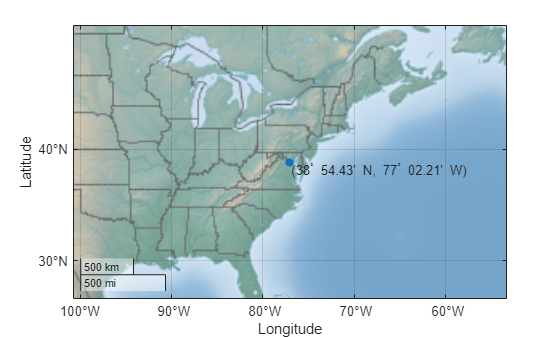# angl2str

Convert angles to character array

## Syntax

``str = angl2str(angles)``
``str = angl2str(angles,signcode)``
``str = angl2str(angles,signcode,format)``
``str = angl2str(angles,signcode,format,n)``

## Description

example

````str = angl2str(angles)` converts numeric angles in degrees to a character array that represents the angles. This function is useful for displaying angles as text on maps.```

example

````str = angl2str(angles,signcode)` returns a character array that represents the angles using the signs or directions specified by `signcode`.```

example

````str = angl2str(angles,signcode,format)` returns a character array that formats the angles as degrees, degrees-minutes, degrees-minutes-seconds, or radians. The function does not convert degrees to radians or vice-versa.```

example

````str = angl2str(angles,signcode,format,n)` specifies the number of decimal places to use in the character array.```

## Examples

collapse all

Convert numeric angles to a character array. By default, the `angl2str` function formats the angles as degrees.

```angles = [-9.7 12.95 24]; str = angl2str(angles)```
```str = 3x16 char array ' - 9.70^{\circ} ' ' 12.95^{\circ} ' ' 24.00^{\circ} ' ```

Convert the same angles to a character array and prefix a plus sign (`+`) to the positive angles.

`strPlus = angl2str(angles,"pm")`
```strPlus = 3x16 char array ' - 9.70^{\circ} ' ' +12.95^{\circ} ' ' +24.00^{\circ} ' ```

Convert the coordinates of Washington, DC to a character array that uses degrees-minutes. Remove the leading and trailing whitespaces.

```lat = 38.9072; lon = -77.0369; latStr = angl2str(lat,"ns","degrees2dm"); latStr = strtrim(latStr); lonStr = angl2str(lon,"ew","degrees2dm"); lonStr = strtrim(lonStr); coordStr = "(" + latStr + ", " + lonStr + ")";```

Display the location and character array on a map.

```geoscatter(lat,lon,"filled") text(lat,lon,coordStr,VerticalAlignment="top")```Convert angles in degrees to a character array. Round the angles to the nearest tenth.

```angles = [8.523 -34.79 -89]; str = angl2str(angles,"none","degrees",-1)```
```str = 3x15 char array ' 8.5^{\circ} ' ' -34.8^{\circ} ' ' -89.0^{\circ} ' ```

## Input Arguments

collapse all

Angles in degrees or radians, specified as a numeric array.

Signs or directions to use in the character array, specified as one of these options:

• `"ew"` — Input angles are longitudes. The character array indicates positive longitudes using `E` and negative longitudes using `W`.

• `"ns"` — Input angles are latitudes. The character array indicates positive latitudes using `N` and negative latitudes using `S`.

• `"pm"` — The character array indicates positive angles using a plus sign (`+`) and negative angles using a minus sign (`-`).

• `"none"` — The character array indicates negative angles using a minus sign (`-`) and does not use a plus sign (`+`) for positive angles.

Data Types: `char` | `string`

Format to use in the character array, specified as one of these options.

OptionFormatExample
`"degrees"`Degrees
`angl2str(31.26,"none","degrees")`
```ans = ' 31.26^{\circ} '```
`"degrees2dm"`Degrees-minutes (DM)
`angl2str(31.26,"none","degrees2dm")`
```ans = ' 31^{\circ} 15.60' '```
`"degrees2dms"`Degrees-minutes-seconds (DMS)
`angl2str(31.26,"none","degrees2dms")`
```ans = ' 31^{\circ} 15' 36.00" '```
`"radians"`Radians
`angl2str(pi/6,"none","radians",-5)`
```ans = ' 0.52360 R '```

The value `^{\circ}` is the LaTeX representation of `°`.

Data Types: `char` | `string`

Number of digits to include in the character array, specified as an integer. When you specify `n`, the `angl2str` function rounds `angles` to the nearest multiple of 10n.

The sign convention for this argument is opposite to the one used by the `round` function.

Example: `angl2str(31.467,"none","degrees",-2)` returns ```' 31.47^{\circ} '```, which is `31.467` rounded to the nearest hundredth.

Example: `angl2str(31.467,"none","degrees",0)` returns ```' 31^{\circ} '```, which is `31.467` rounded to the nearest integer.

## Output Arguments

collapse all

Text representation of the input angles, returned as a character array.

Data Types: `char`

## Version History

Introduced before R2006a